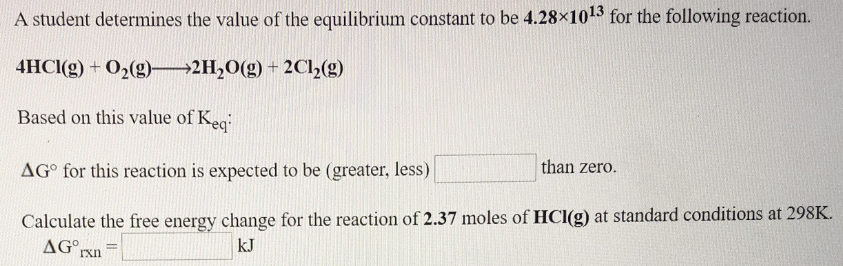# A student determines the value of the equilibrium constant to be 4.28x10^13 for the following reaction. 4HCl(g) + O2(g) → 2H2O(g) + 2Cl2(g) Based on this value of Keq: ΔG° for this reaction is expected to be (greater, less)_______ than zero. Calculate the free energy change for the reaction of 2.37 moles of HCl (g) at standard conditions at 298K.